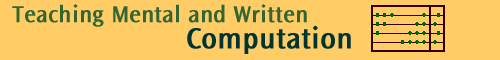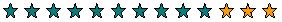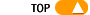Back to Multiply

# Distributive property of multiplication over addition

The distributive property of multiplication over addition explains how multiplication and addition interact. It simplifies many multiplication questions. The formal algorithm and many informal algorithms are based on this property.

The distributive property of multiplication over addition is illustrated by the following examples.

Example 1

The diagram shows that 3 groups of 4 yellow stars and 3 groups of 2 blue stars can be considered as 3 groups of 6 stars.3 groups of 4 + 3 groups of 2 = 3 groups of 6

i.e. (3 x 4) + (3 x 2) = 3 x (4 + 2)

Example 2

4 x 13 is 4 groups of 13

Since 13 = 10 + 3, 4 groups of 13 will be 4 groups of 10 + 4 groups of 3

4 groups of 134 groups of 10 and 4 groups of 3We can use this to calculate 4 x 13 from tables up to ten tens:

4 x 13 = (4 x 10) + (4 x 3) = 40 + 12 = 52

General statement

For any three numbers a, b and m,

m x (a + b) = (m x a ) + (m x b)

This is called the distributive property of multiplication over addition.

Because multiplication is commutative (the order doesn't matter), the distributive property also says that:

(a + b) x m = (a x m ) + (b x m)